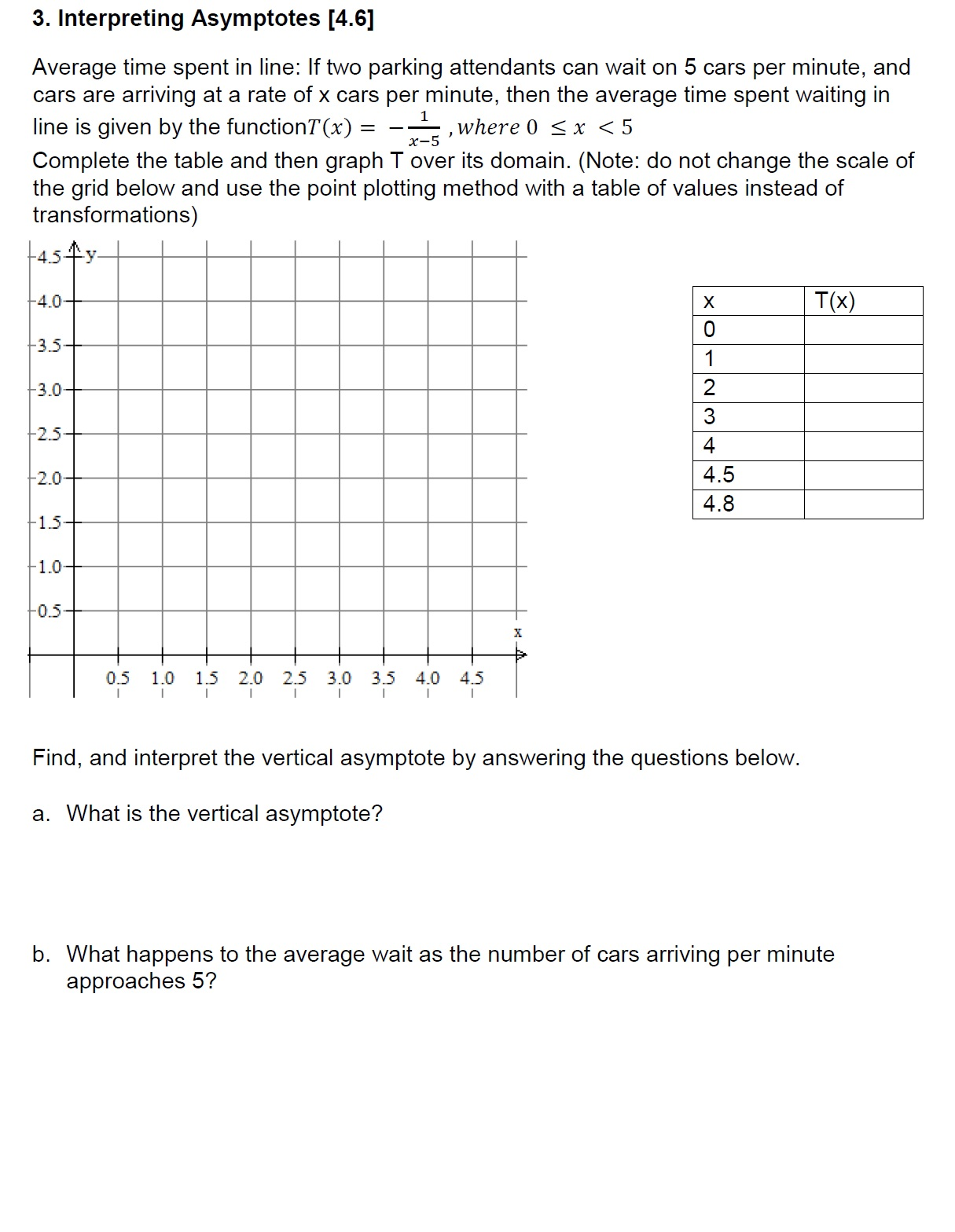# 3. Interpreting Asymptotes [4.6]Average time spent in line: If two parking attendants can wait on 5 cars per minute, andcars are arriving at a rate of x cars per minute, then the average time spent waiting inline is given by the functionT (x)Complete the table and then graph T over its domain. (Note: do not change the scale ofthe grid below and use the point plotting method with a table of values instead oftransformations), where 0

Question
6 viewshelp_outlineImage Transcriptionclose3. Interpreting Asymptotes [4.6] Average time spent in line: If two parking attendants can wait on 5 cars per minute, and cars are arriving at a rate of x cars per minute, then the average time spent waiting in line is given by the functionT (x) Complete the table and then graph T over its domain. (Note: do not change the scale of the grid below and use the point plotting method with a table of values instead of transformations) , where 0
check_circle

Step 1

Complete the table as follows.

Step 2

Plot the graph of T(x) as follows.

Step 3

(a) The vertical asymptote i

The vertical asymptote is a vertical l...

### Want to see the full answer?

See Solution

#### Want to see this answer and more?

Solutions are written by subject experts who are available 24/7. Questions are typically answered within 1 hour.*

See Solution
*Response times may vary by subject and question.
Tagged in

### Algebra